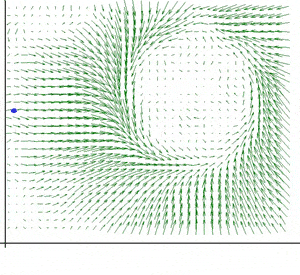# Line Integral Visualization

Hello Everyone
I have a few questions regarding line integrals. First what are they? What is the difference between them and the normal integrals? For eg, The normal integrals can be easily understood by visualizing the area bounded by them, in the same way is there any way as to visualize the line integrals as we perform the integration operation, in lay man's terms?

I found that they are used in Gaussian electric fields, Why are they used here?, I mean why can't we use the normal integral in such problems?
what property of the line integral advocates the use of them in such type of problems?

I had Googled a bit and also referred to old threads on this topic in PF, but couldn't understand.Thats why i'm creating a new thread

Line integral continuously adds up values of a function along a curve

For example, suppose that a scalar function describes temperature distribution in a plane, call it T(x, y) = x + y

T(1,1) = 2 degrees, at T(.5, 0) = 0.5 degrees, at T(0.01, 0.02) = 0.003

and so on, you are just evaluating the function,

if those points were passing through some curve, the line integral would add them up

2 + 0.5 + 0.003

and so on

Hmm.. when you say normal integration, from your example of a normal integral i assume u mean finding the area under a 2D curve, like y=x2 between a pair of bounds.

line integration is used when instead of having a function of 1 variable (like f(x) = x2) you have a function of 2 or more variables, e.g. f(x,y) = x2 + y2 and you wanted to find the area in a slice of it rather than the total volume of the solid this function generates.

I am unfamiliar with the maths of gaussian electiric feilds so i can't comment on that.

Cheers. Visualisation is always good.Last edited by a moderator:
Thank you all for your replies, Now that the concept of line integral is clear,I have another doubt. When we consider a closed line integral, what is the area that we are evaluating?
Is it the area enclosed between the curves, I doubt it.

Draw a closed line integral and study it: Take a function of two variables f(x,y) which is nicely positive in the first quadrant. Now, draw a circle in the x-y plane in the first quadrant. Now, here it the tricky part. Draw the cylinder that connects the circle, straight up to the surface of f(x,y). Can you visualize that? The cylinder end sitting on the x-y plane is of course nice and flat while the end touching f(x,y) will in general not be flat but will follow the surface of f(x,y) along it's surface where the cylinder meets it. Then:

$$\mathop\oint\limits_{\substack{\text{my}\\ \text{circle}}}f(x,y) ds$$

is the surface area of that cylinder. Post a picture, transparent so that we can see where everything is touching then explain to us why:

$$\mathop\oint\limits_{\substack{\text{my}\\ \text{circle}}}f(x,y) ds=\lim_{\|\Delta \|} \sum_{i} f(u_i,v_i) \Delta s_i$$

Do something else, compute that closed line integral using just a (finite) Riemann sum above, say for 360 elements and then compare it to the actual value of the integral. Should be close for well-behaved functions, say 2 or 3 decimal places maybe.

@ Squidsoft, Thanks for the reply. I understand the cylindrical area part. i did a little research on the topic and found another interesting example for explaining this closed line integral. It has similar views based on Waht's reply.

For example, suppose that a scalar function describes temperature distribution in a plane, call it T(x, y) = x + y

T(1,1) = 2 degrees, at T(.5, 0) = 0.5 degrees, at T(0.01, 0.02) = 0.003

and so on, you are just evaluating the function,

if those points were passing through some curve, the line integral would add them up

2 + 0.5 + 0.003

From my little research on Closed line integral i found that if these points (Points from the curve) are on a circle which is a close figure then the integral that we evaluate is the closed integral.

The cylinder end sitting on the x-y plane is of course nice and flat while the end touching f(x,y) will in general not be flat but will follow the surface of f(x,y) along it's surface where the cylinder meets it. Then:

is the surface area of that cylinder

Does my little research is same as the above explanation that you gave? Are they both one and the same? Correct me if its not.